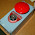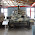## Sunday, 5 October 2014

### Penetration Equations

When designing a new gun, it is hard to know how much armour its shells will penetrate without building it. Similarly, if all you know is how thick enemy armour is but do not have a sample to shoot at, you will not know if you can penetrate it with your existing guns, or if need to develop new ones. These two problems predate tank warfare, and arose when the first armoured warships hit the seas. An equation was created by Commandant Jacob de Marre to estimate the thickness of armour a shell will penetrate, given the penetration data of another shell. WWII Ballistics - Armor and Gunnery defines the equation as follows.

Naturally, the equation is only an estimate (the book then goes on to present many cases where the equation doesn't work and requires modification of exponents). It works best when a similar shell is chosen, so try to find a reference shell of a similar caliber and type from the same manufacturer.

Another way to estimate penetration is the Krupp formula, also designed during the era of battleships. This formula is defined as follows:

Where B is the thickness of armour penetrated in decimeters, V is the velocity of the shell on impact, P is the mass of the projectile, and D is the projectile caliber. K is a resistance constant, which represents the quality of the armour and the capacity of the shell to penetrate it. When in doubt, use the value of 2400, which was the value for standard quality armour used in Soviet calculations.

However, since doing math by hand is for grade schoolers, I would like to introduce a handy utility: a Javascript penetration calculator. You can use both formulas to get a quick estimate of how much armour a shell should penetrate.

For example, if I plug in the data for a Soviet 45 mm model 1937 anti-tank gun (45 mm caliber, 1.43 kg mass, 760 m/s muzzle velocity) into the Krupp calculator, I get a result of 56 mm. Soviet penetration tables give the gun 57 mm of penetration, so that's a pretty good estimate! Now, to estimate the penetration of a model 1942 gun that uses the same shell, all I have to do is copy the values to the reference section and up the estimate's velocity to 870 m/s, which gives me a result of 68 with the penetration in Soviet tables being 69 mm. Again, only one millimeter off! Krupp's estimate for the 45 mm mod. 1942 gun is 65 mm, which isn't too bad.

Another example, with the D-25 gun. Plugging in the values (122 mm shell, 25 kg mass, 800 m/s) gives 151 mm of penetration, which is a bit lower than the Soviet value of 165 mm. When I copy over the data to calculate the penetration of the BL-9 gun (muzzle velocity of 1000 m/s), I get 227 mm, which is close to the value in the penetration table (230 mm). However, Krupp's estimate only gives 189 mm, which is quite far off.

As you can see, using the DeMarre equation is likely to give you much better numbers, but Krupp can also serve as a ballpark estimate when you have nothing to compare with.

1.This is ridiculously handy, a thanks a million.

2.Nice article. Which one used the Soviets for their calculations?

3.This is quite cool, greatest of thanks.

4.How would you take into account alternative shell materials - i.e., APCR with tungsten carbide?

1.There might be an alternate K value to use with APCR, but I haven't looked into it.

5.Great post, hugely useful, but I can only make the units balance in the Krupp equation if I use the square-root of tensile strength for K rather than tensile strength itself. Thus, I think K should be the square-root of 2400 for steel. Am I missing something here?

Thanks,
George Young

6.Can some one tell me which one will be taken as basic armour equation for penetration

7.At what range is the shell hitting the target?

8.At what range would the value be for? Is there any way to determine drop-off of penetration over a given distance?

1.It depends on the gun and its shell. You will need to find the ballistics tables and get the impact velocity from there.

9.I don't know if this works well for APFSDS. It looks like it underestimates the penetration of an Abrams shell by a lot.

10.I built a DeMarre calculation program that uses the angle as well as different K factors. Demarre doesn't give good results beyond 30°.
Try this 57mm Wt. 3.14 kg K factor=2400 velocity: 973.8 m/s.

11.I have designed and programmed my own python calculator version of this, and it includes if the armour is sloped or not and if it will penetrate that said armour. Do you want to see it?